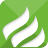# recat示例 - 数据驱动模板及交互获取数据完整流程

2013-01-14 11:28:33 | 浏览量：608 react webpack es6```this.state = {
// selet项初始化数据
selectData: [{ size: 175, color: 'white' }]
}```

`160165170175180185`

react需要手动处理的，触发事件后，获取选择的值，改动对应state值。因为是数组，要根据数组下标index来找到对应的数据，这里的index在渲染的时候以data-index属性加入到select元素中。在改数据时可以判断下type，区别下size和color两个元素，这样可以公用。

```setSelectData = (e) => {
let value = e.target.value;
var index = parseInt(e.target.getAttribute("data-index"));
let type = e.target.getAttribute("data-type");

var lists = this.state.selectData;
var presentItem = lists[index];

if (type == 'size') {
presentItem.size = parseInt(value);
} else if (type == 'color') {
presentItem.color = value;
}

this.setState({ selectData: lists })
}```

```addItem = (e) => {
var index = parseInt(e.target.getAttribute("data-index"));
var listsNew = this.state.selectData;
var presentItem = listsNew[index];
listsNew.splice(index, 0, presentItem)
this.setState({ selectData: listsNew })
}``````addItem = (e) => {
var index = parseInt(e.target.getAttribute("data-index"));
var listsNew = Common.deepClone(this.state.selectData);
var presentItem = Common.deepClone(listsNew[index]);//深拷贝
listsNew.splice(index, 0, presentItem)
this.setState({ selectData: listsNew })
}```

``` deepClone(data) {
let type = this.getType(data);
let obj;
if (type === 'array') {
obj = [];
} else if (type === 'object') {
obj = {};
} else {
// 不再具有下一层次
return data;
}
if (type === 'array') {
for (let i = 0, len = data.length; i < len; i++) { obj.push(this.deepClone(data[i])); } } else if (type === 'object') { for (let key in data) { obj[key] = this.deepClone(data[key]); } } return obj; }```0.4644s